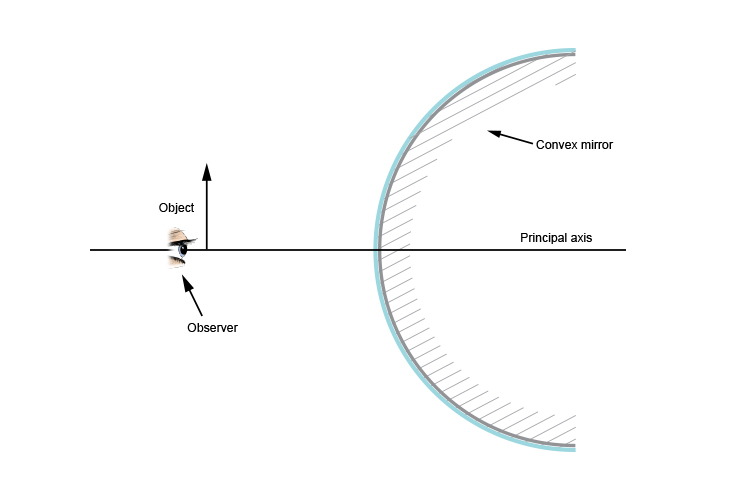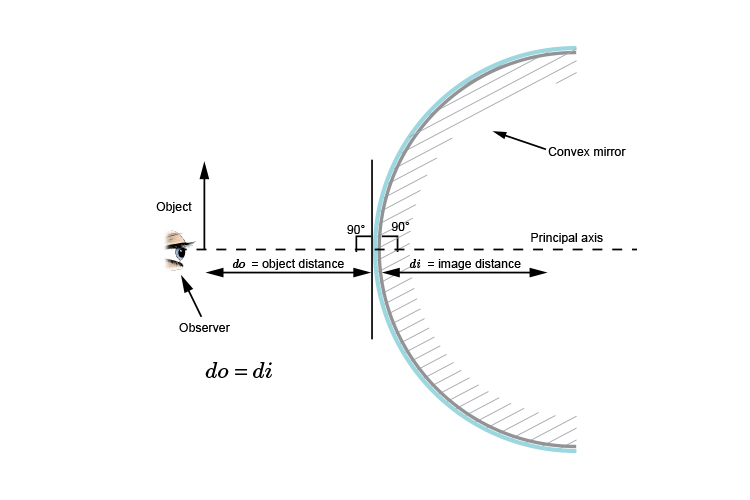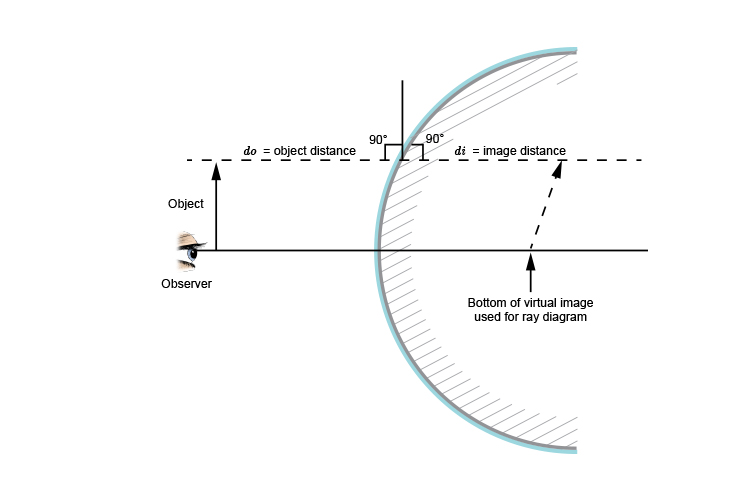# Using flat mirror ray diagram rules for convex mirrors

When we observe an object in a convex mirror there are three ways to try to work out how the light rays from an object focus into the eye of an observer:

1. Use the ray diagram flat/plane mirror rules
2. Use the laws of reflection
3. Use new ray diagram rules and create a virtual image

Here we shall discuss method 1, trying to use the ray diagram flat/plane mirror rules which are:

Behind the mirror
Equal distance
Same size

The following diagram shows a convex mirror, an object and an observer:The first stage would be to draw an image on the other side of the mirror perpendicular (at 90°) to the mirror.

This is easy for the one end of the arrow that is on the principal axis and in line with the observer. (see below)We can mark on the principal axis an image distance that is equal to the object distance.

For the top of the arrow things get very complicated and unclear.If we apply the same rules that the image is the same distance behind the mirror we can immediately see there are problems.

The virtual image used to work out the light ray lines from the object to the observer is sloped.

Although these rules work for flat mirrors they definitely don't work for convex mirrors.